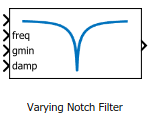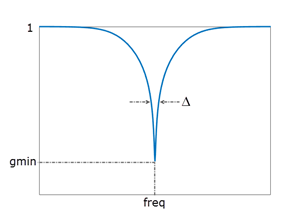# Varying Notch Filter

Notch filter with varying coefficients

• Library:
• Control System Toolbox / Linear Parameter Varying

•## Description

The block implements a continuous-time notch filter with varying coefficients. The instantaneous transfer function of the filter is given by:

`$N\left(s\right)=\frac{{s}^{2}+2*gmin*damp*freq*s+fre{q}^{2}}{{s}^{2}+2*damp*freq*s+fre{q}^{2}},$`

where gmin, damp, and freq are the values supplied at the corresponding input ports. These parameters control the notch depth and frequency as shown in the following illustration. The damping ratio damp controls the notch width Δ; larger damp means larger Δ.Use this block and the other blocks in the Linear Parameter Varying library to implement common control elements with variable parameters or coefficients. For more information, see Model Gain-Scheduled Control Systems in Simulink.

## Ports

### Input

expand all

Notch filter input signal

Value of the notch frequency, specified in rad/s.

Value of the gain at notch frequency, in absolute units. This value controls the notch depth. The notch filter has unit gain at low and high frequency. The gain is lowest at the notch frequency, the value at the freq port.

Value of the damping ratio, specified as a positive scalar value. The damping ratio controls the notch width; the closer to 0, the steeper the notch.

### Output

expand all

Notch filter output signal.

## Version History

Introduced in R2017b